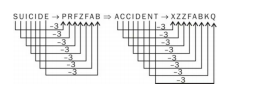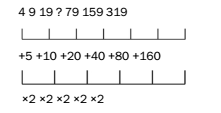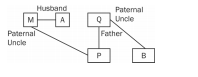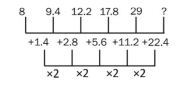# RRB ALP 2018 Practice Test Papers | Reasoning Questions (Day-22)

Dear Aspirants, Here we have given the Important RRB ALP & Technicians Exam 2018 Practice Test Papers. Candidates those who are preparing for RRB ALP 2018 can practice these Reasoning Questions to get more confidence to Crack RRB 2018 Examination.

[WpProQuiz 1802]

Click “Start Quiz” to attend these Questions and view Solutions

1. Identify the odd one out.

(A) Carbon dioxide

(B) Methane

(C)Sulphur dioxide

(D) Ozone

1. Choose the odd one out.

(A) Binocular

(B) Mirror

(C) Magnifier

(D) Microscope

1. Which of the following combinations of mathematical signs can correctly replace ‘*’ from 28 * 32 * 8 * 2 * 3 * 26?

(A) +–×÷=

(B) –+×÷=

(C) +–÷×=

(D) +÷–×=

1. In a certain coding system, if SUICIDE is coded as PRFZFAB, what will be the code for ACCIDENT?

(A) XYYFABKQ

(B) XZZFABJR

(C) XZZFABKQ

(D) ZZZFABKQ

1. 14: 9:: 26:?

(A) 12

(B) 13

(C) 31

(D) 15

1. 4, 9, 19,?, 79, 159, 319

(A) 59

(B) 39

(C) 29

(D) 49

1. 125, 171, 263, 401, 585,?

(A) 835

(B) 815

(C) 792

(D) 788

1. Select the set of letters from the given alternatives which, when sequentially placed at the gaps in the given letter series, completes it.

a_ab_a_aabb_aaabba_aabbaaa_bba

(A)ababab

(B)ababaa

(C)aaaaaa

(D)abaaaa

1. P’s father Q is B’s paternal uncle and A’s husband M is P’s paternal uncle. How is A related to B?

(A) Mother

(B) Sister

(C) Daughter

(D) None of these

1. 8, 9.4, 12.2, 17.8, 29,?

(A) 53.6

(B) 51.4

(C) 52.1

(D) 48.6

Carbon dioxide, methane and ozone are greenhouse gases and prevent heat from escaping the atmosphere. These gases increase the temperature of the Earth. Carbon and methane are major greenhouse gases.

All others magnify the image of an object, except mirror.

28 + 32 ÷ 8 – 2 × 3 = 28 + 4 – 6 = 28 – 2 = 265. Answer: (D)

14 = (2 × 9 – 4)

26 = (2 × 15 – 4)

Therefore, ? = 1519+20=39

125 + 46 = 171

171+ (46 × 2) = 263

263 + (46 × 3) = 401

401 + (46 × 4) = 585

585 + (46 × 5) = 815

The given series is formed by repeating the set ‘aaabba’. So, option 4 will complete the series.10. Answer: (B)

The number in place of?should be: 29 +.22.4 = 51.4.RRB ALP 2018 Practice Test Papers | Reasoning Questions (Day-1)

RRB ALP 2018 Practice Test Papers | Reasoning Questions (Day-2)

RRB ALP 2018 Practice Test Papers | Reasoning Questions (Day-3)

RRB ALP 2018 Practice Test Papers | Reasoning Questions (Day-4)

RRB ALP 2018 Practice Test Papers | Reasoning Questions (Day-5)

RRB ALP 2018 Practice Test Papers | Reasoning Questions (Day-6)

RRB ALP 2018 Practice Test Papers | Reasoning Questions (Day-7)

RRB ALP 2018 Practice Test Papers | Reasoning Questions (Day-8)

RRB ALP 2018 Practice Test Papers | Reasoning Questions (Day-9)

RRB ALP 2018 Practice Test Papers | Reasoning Questions (Day-10)

RRB ALP 2018 Practice Test Papers | Reasoning Questions (Day-11)

RRB ALP 2018 Practice Test Papers | Reasoning Questions (Day-12)

RRB ALP 2018 Practice Test Papers | Reasoning Questions (Day-13)

RRB ALP 2018 Practice Test Papers | Reasoning Questions (Day-14)

RRB ALP 2018 Practice Test Papers | Reasoning Questions (Day-15)

RRB ALP 2018 Practice Test Papers | Reasoning Questions (Day-16)

RRB ALP 2018 Practice Test Papers | Reasoning Questions (Day-17)

RRB ALP 2018 Practice Test Papers | Reasoning Questions (Day-18)

RRB ALP 2018 Practice Test Papers | Reasoning Questions (Day-19)

RRB ALP 2018 Practice Test Papers | Reasoning Questions (Day-20)

RRB ALP 2018 Practice Test Papers | Reasoning Questions (Day-21)